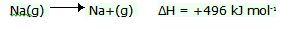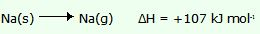# lionization enthalpy

The ionization energy (IE) is qualitatively defined as the amount of energy required to remove the most loosely bound electron, the valence electron, of an isolated gaseous atom to form a cation. It is quantitatively expressed in symbols as:

X + energy → X+ + e

"The enthalpy of formation is the energy change when 1 mole of a substance is formed from its constituent elements in their standard states"

• The elements are in their usual states under standard conditions. i.e at 25ºC and 1 atmosphere pressure (100 kPa).

• The enthalpy of formation of an element in its standard state is zero by this definition.

• The energy is given per mole of substance.

This is defined as the energy required to remove 1 mole of electrons from 1 mole of gaseous particles producing 1 mole of ions. It can be stated as 1st ionisation energy, 2nd ionisation energy etc.

The 1st ionisation energy is the energy required to remove 1 mole of electrons from 1 mole of gaseous atoms to produce 1 mole of singly charged positive ions. It is always endothermic.

### Key points to remember are:

• The value is quoted per mole of species

• The particles must be gaseous

### Example: The first ionisation energy of sodiumEnthalpy of atomisation

This is the energy needed to transform an element in its standard state into 1 mole of gaseous atoms. It is always endothermic.

### Example: The enthalpy of atomisation of sodiumLattice enthalpy

This is possible to define from two points of view

• Forming of 1 mole of a crystal lattice from gaseous ions (exothermic)

• Separating 1 mole of a crystal lattice into gaseous ions at infinite separation (endothermic).

The way you define it is not important providing that you understand the consequences for the enthalpy change in terms of the exo- and endothermic processes.

### Example: The lattice enthalpy of sodium chloride

Na+(g) + Cl-(g) = NaCl(s) ΔH = -786 kJ mol-1